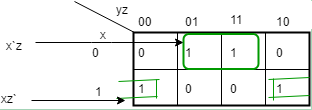# UGC NET COMPUTER SCIENCE SOLVED PAPERS 2014-16 - UGC NET Computer Science Paper 2 JULY 2016

>>>>>>>>UGC NET Computer Science Paper 2 JULY 2016

• A

1 ⊕ 0 = 1• B

1 ⊕ 1 ⊕ 1 = 1• C

1 ⊕ 1 ⊕ 0 = 1• D

1 ⊕ 1 = 0• Option : C
• Explanation :
We know that x ⊕ y = x`y + xy` So,
1. 1 ⊕ 0 = 1`0 + 10` = 0 * 0 + 1 * 1 = 0 + 1 = 1
2. 1 ⊕ 1 ⊕ 1 = (1 ⊕ 1) ⊕ 1 = (1`1 + 11`) ⊕ 1 = (0) ⊕ 1 = 1`0 + 10` = 0 * 0 + 1 * 1 = 0 + 1 = 1
3. 1 ⊕ 1 ⊕ 0 = 1 ⊕ (1 ⊕ 0 ) = 1 ⊕ ( 1`0 + 10` = 0 * 0 + 1 * 1 = 0 + 1 ) = 1 ⊕ 1 = 1`1 + 11` = 0
4. 1 ⊕ 1 = 1`1 + 11` = 0
So, option (C) is correct.

• A

16• B

32• C

48• D

64• Option : D
• Explanation :
The IEEE-754 double-precision format to represent floating point numbers, has a length of 64 bits. 1 bit for sign, 11 bit for exponent and rest 52 is for fraction. So, option (D) is correct.

 x y z F 0 0 0 0 0 0 1 1 0 1 0 0 0 1 1 1 1 0 0 1 1 0 1 0 1 1 0 1 1 1 1 0

• A

F=yz'+ y'z• B

F=xy'+x'y• C

F=x'z+xz'• D

F=x'z+xz'+xyz• Option : C
• Explanation :So, option (C) is correct.

• A

AB­'• B

AB­'C• C

A'B• D

ABC• Option : A
• Explanation :
(AB` + AB`C + AC) (A`C` + B`) = AB` +AB`C +AB`C = AB` +AB`C = AB`(1 + C) = AB` So, option (A) is correct.

• A

No change• B

Set• C

Reset• D

Toggle